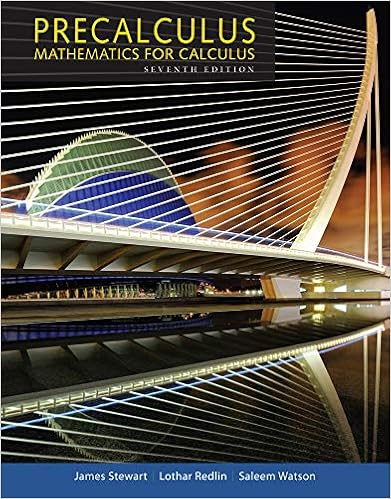# Math 105 Exam 3.docx - Q1 The graph of a logarithmic...

• Test Prep
• 17
• 100% (26) 26 out of 26 people found this document helpful

This preview shows page 1 - 3 out of 17 pages.

##### We have textbook solutions for you!
The document you are viewing contains questions related to this textbook.The document you are viewing contains questions related to this textbook.
Chapter 4 / Exercise 58
Precalculus: Mathematics for Calculus
Redlin/StewartExpert Verified
Q1. The graph of a logarithmic function is shown. Select the function which matches the graph. a. y = log 2(x + 1)b. y = log 2(x - 1)c. y = log 2d. y = log 2x - 1
x
Q2. Express 6log bm - log bn as a single logarithm.
n
Q3. Find the domain of the composite function f g if f(x) = 28/x and g(x) = -8/(x - 7).
Q4. A size 6 dress in Country C is size 52 in Country D. A function that converts dress sizes
##### We have textbook solutions for you!
The document you are viewing contains questions related to this textbook.The document you are viewing contains questions related to this textbook.
Chapter 4 / Exercise 58
Precalculus: Mathematics for Calculus
Redlin/StewartExpert Verified
in Country C to those in Country D is f(x) = 2(x + 20). Find a formula for the inverse of the function described.
Q5. In 1990, the population of a country was estimated at 4 million. For any subsequent year the population, P(t) (in millions), can be modeled by the equation P(t) = 240/(5 + 54.99e-0.0208t), where t is the number of years since 1990. Estimate the year when the population will be 21 million.a. approximately the year 2093b. approximately the year 2041c. approximately the year 2088d. approximately the year 2016
Q6. Write log 7√(xy)/15 as the sum and/or difference of logarithms. Express powers as factors.
15
715
15
15Q7. Find the domain of the function f(x) = log 5(100 - x2).
)
•••